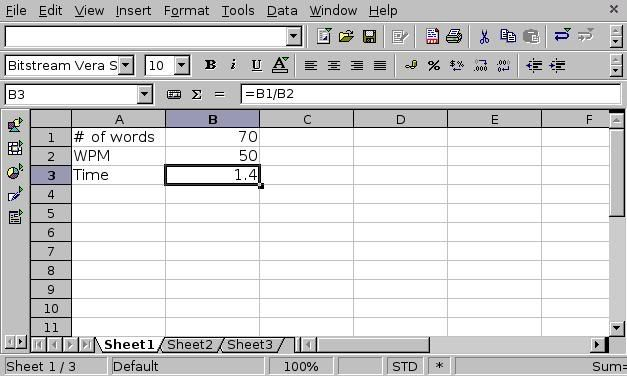## Recommended Posts

I was just wondering what is the formula for words per minute and how to put it in a spreadsheet??

I my spreadsheet is like this so far:

A1= # of Words    B1= X

A2= WPM            B2= X

A3= Time            B3= Formula???

So B1, and B2 is the # of words and WPM.....

If anyone could tell me what to put in B3, that would be great!

dk##### Share on other sites
I was just wondering what is the formula for words per minute and how to put it in a spreadsheet??

The formula is, uh, words / minutes. How you handle the spreadsheet with depend on what software you're using and how you want to format the sheet. Here's an example I made in OpenOffice:The equation for cell B3 is in the equation bar.

##### Share on other sites

To get seconds you'd need to multiply the minutes by 60 to get seconds, then use modulus 60 to get the number of seconds.

Something like:

seconds = (time * 60) % 60

minutes = int(time / 60) providing int rounds down, otherwise you may need to do a floor(time) to eliminate the decimal

then minutes:seconds would be your output.

Hope that helps.